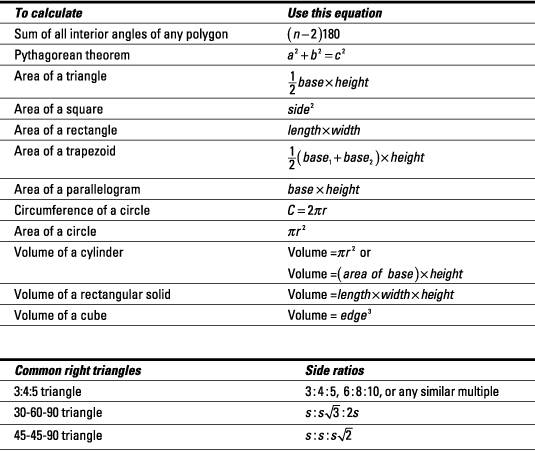##### GRE 1,001 Practice Questions For DummiesSuccess on the GRE’s Quantitative Reasoning section has a great deal to do with applying logic and common sense. Although the computerized GRE provides a calculator, you can save time by reserving it for complex math, not basic arithmetic you should know. Here are some tips for doing your best on the Quantitative section of the test.

## Memorizing common equations

Memorize the following equations before test day, because the GRE doesn’t provide them:

• Work: To solve work problems, in which different people take different amounts of time to complete a task, use the following formula: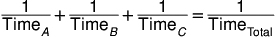• Probability: To find probability, place the number of possible desired outcomes over the number of total possible outcomes: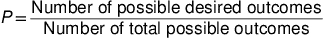• Permutations: To calculate permutations (the number of ways a subset of items or events can be arranged when the order in which they’re arranged matters), use this equation, where n is the total number of items or events and r is the subset of items or events you’re asked to arrange: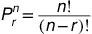• Combinations: To calculate combinations (the number of ways a subset of items can be selected when order doesn’t matter), use this equation, where n is the total number of items or events and r is the subset of items or events you’re asked to arrange: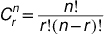## Brushing up on basic statistics

Make sure you can find these common values related to statistics:

• Mean (average): To calculate the mean, total all the values and then divide by the number of values.

• Median: To determine the median, arrange the numbers from smallest to largest; then pick the one in the middle or average the two middle numbers.

• Mode: To determine the mode, arrange the numbers from smallest to largest and identify the number that appears most frequently.

• Range: To calculate the range, subtract the smallest term from the largest term.

• Standard deviation: Estimate using the mean deviation:

1. Find the mean (average).

2. Find the positive distance of each value from the mean.

3. Average the distances from Step 2.

## Gearing up for geometry questions

You’ll encounter geometry on the GRE, so memorize these equations, too: